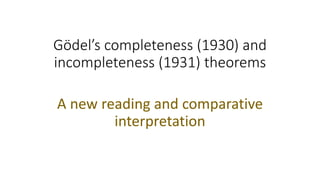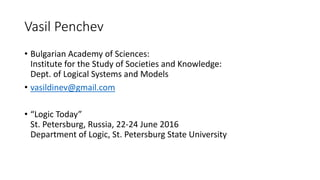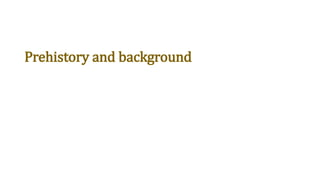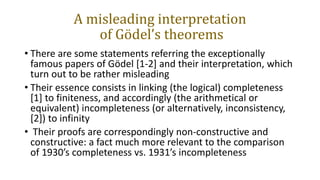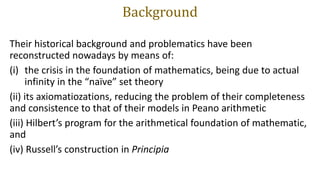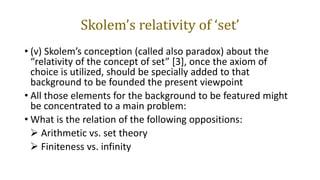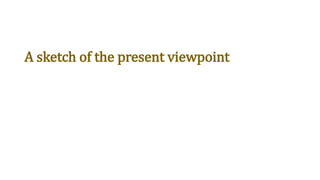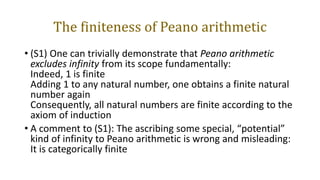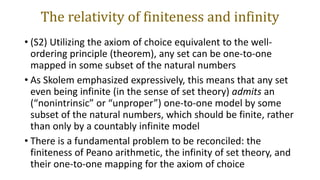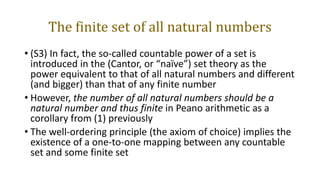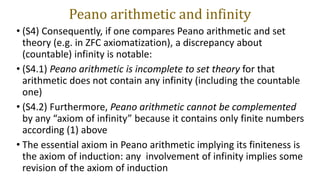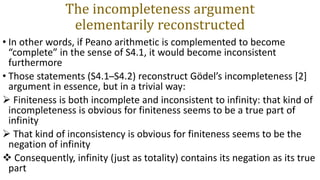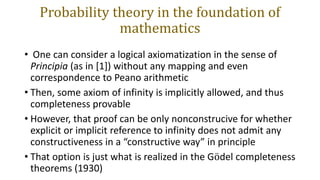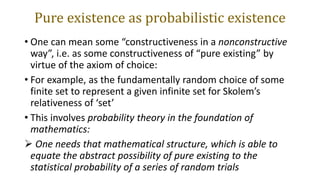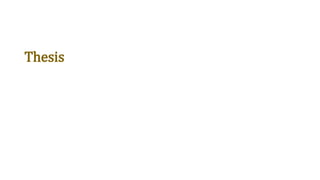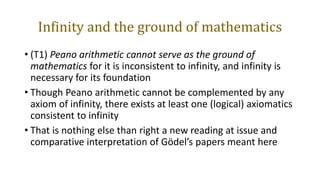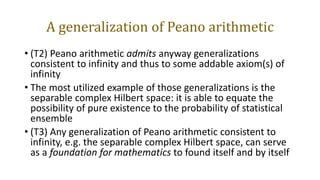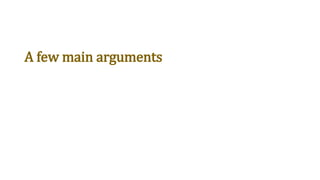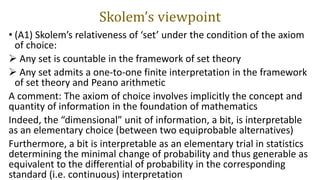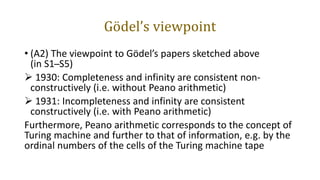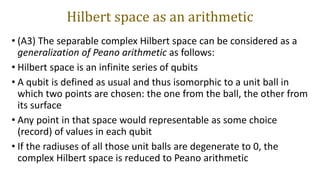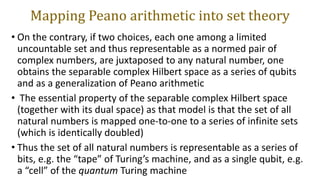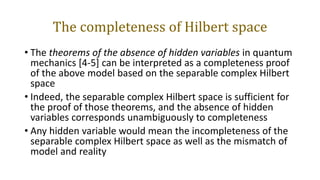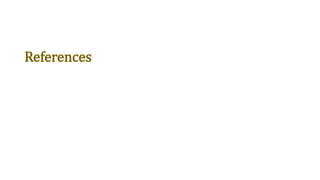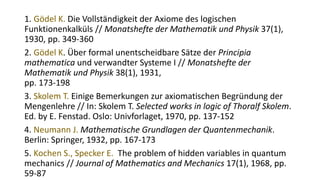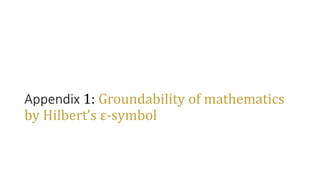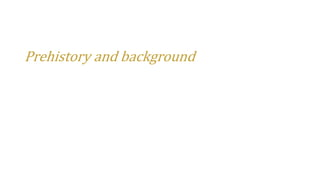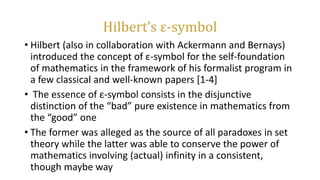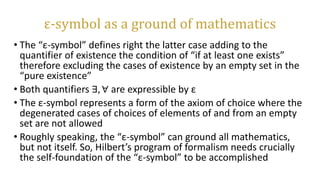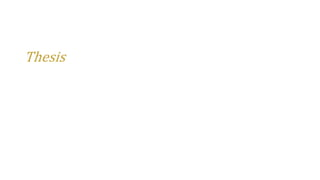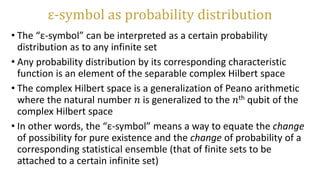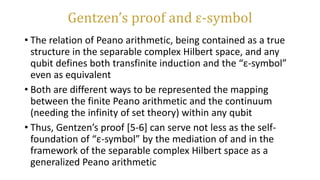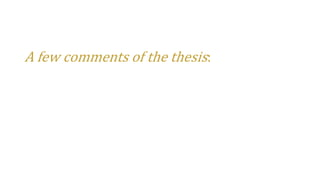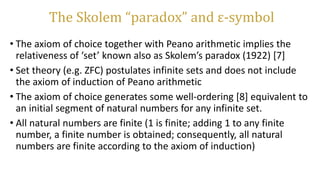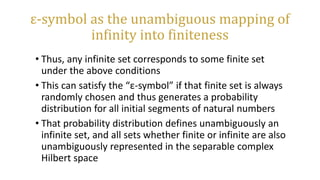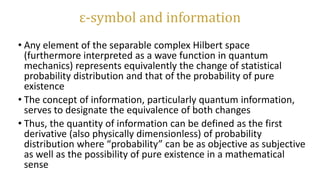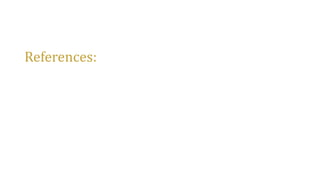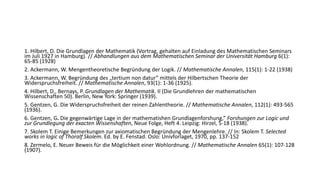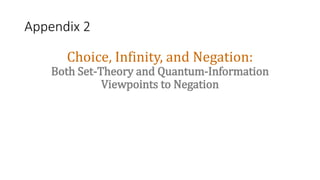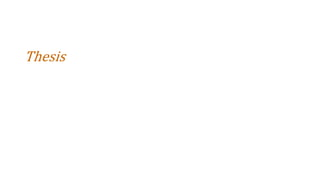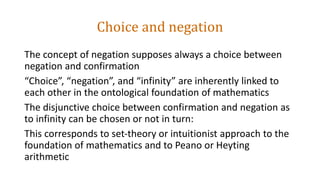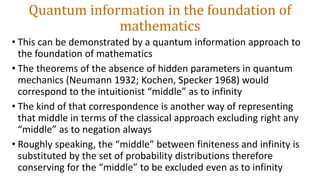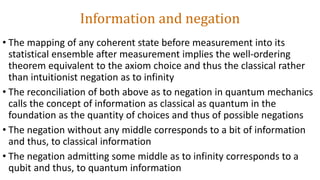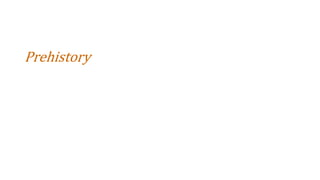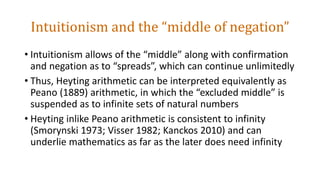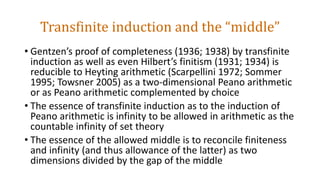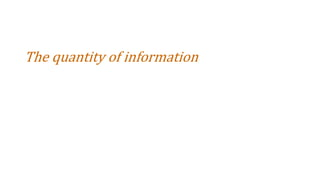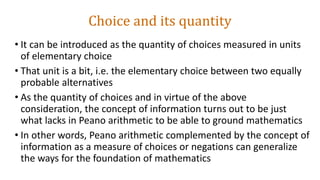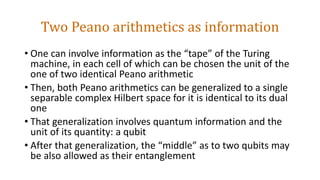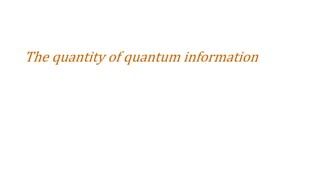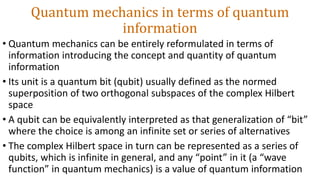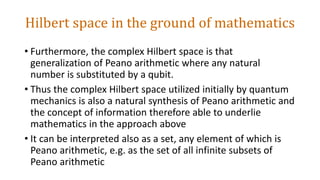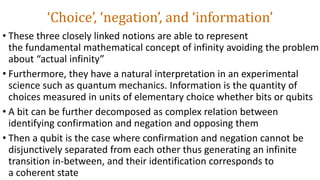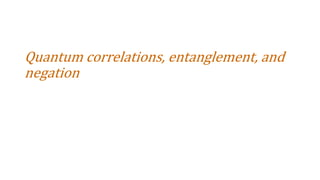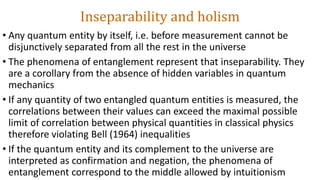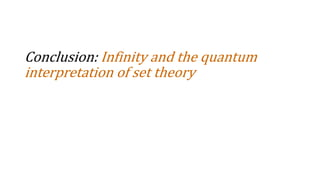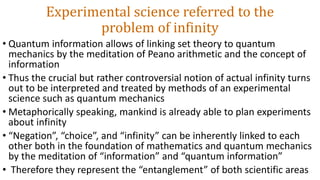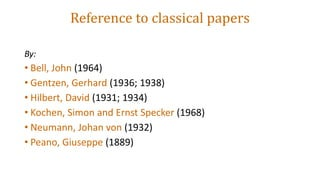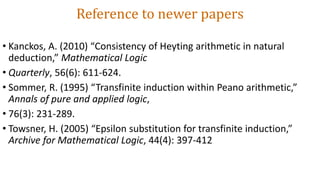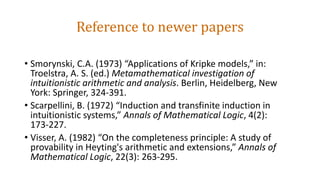1 de 61

### Gödel’s completeness (1930) nd incompleteness (1931) theorems: A new reading and comparative interpretation

1. Gödel’s completeness (1930) and incompleteness (1931) theorems A new reading and comparative interpretation
2. Vasil Penchev • Bulgarian Academy of Sciences: Institute for the Study of Societies and Knowledge: Dept. of Logical Systems and Models • vasildinev@gmail.com • “Logic Today” St. Petersburg, Russia, 22-24 June 2016 Department of Logic, St. Petersburg State University
3. Prehistory and background
4. A misleading interpretation of Gödel’s theorems • There are some statements referring the exceptionally famous papers of Gödel [1-2] and their interpretation, which turn out to be rather misleading • Their essence consists in linking (the logical) completeness  to finiteness, and accordingly (the arithmetical or equivalent) incompleteness (or alternatively, inconsistency, ) to infinity • Their proofs are correspondingly non-constructive and constructive: a fact much more relevant to the comparison of 1930’s completeness vs. 1931’s incompleteness
5. Background Their historical background and problematics have been reconstructed nowadays by means of: (i) the crisis in the foundation of mathematics, being due to actual infinity in the “naïve” set theory (ii) its axiomatiozations, reducing the problem of their completeness and consistence to that of their models in Peano arithmetic (iii) Hilbert’s program for the arithmetical foundation of mathematic, and (iv) Russell’s construction in Principia
6. Skolem’s relativity of ‘set’ • (v) Skolem’s conception (called also paradox) about the “relativity of the concept of set” , once the axiom of choice is utilized, should be specially added to that background to be founded the present viewpoint • All those elements for the background to be featured might be concentrated to a main problem: • What is the relation of the following oppositions:  Arithmetic vs. set theory  Finiteness vs. infinity
7. A sketch of the present viewpoint
8. The finiteness of Peano arithmetic • (S1) One can trivially demonstrate that Peano arithmetic excludes infinity from its scope fundamentally: Indeed, 1 is finite Adding 1 to any natural number, one obtains a finite natural number again Consequently, all natural numbers are finite according to the axiom of induction • A comment to (S1): The ascribing some special, “potential” kind of infinity to Peano arithmetic is wrong and misleading: It is categorically finite
9. The relativity of finiteness and infinity • (S2) Utilizing the axiom of choice equivalent to the well- ordering principle (theorem), any set can be one-to-one mapped in some subset of the natural numbers • As Skolem emphasized expressively, this means that any set even being infinite (in the sense of set theory) admits an (“nonintrinsic” or “unproper”) one-to-one model by some subset of the natural numbers, which should be finite, rather than only by a countably infinite model • There is a fundamental problem to be reconciled: the finiteness of Peano arithmetic, the infinity of set theory, and their one-to-one mapping for the axiom of choice
10. The finite set of all natural numbers • (S3) In fact, the so-called countable power of a set is introduced in the (Cantor, or “naïve”) set theory as the power equivalent to that of all natural numbers and different (and bigger) than that of any finite number • However, the number of all natural numbers should be a natural number and thus finite in Peano arithmetic as a corollary from (1) previously • The well-ordering principle (the axiom of choice) implies the existence of a one-to-one mapping between any countable set and some finite set
11. Peano arithmetic and infinity • (S4) Consequently, if one compares Peano arithmetic and set theory (e.g. in ZFC axiomatization), a discrepancy about (countable) infinity is notable: • (S4.1) Peano arithmetic is incomplete to set theory for that arithmetic does not contain any infinity (including the countable one) • (S4.2) Furthermore, Peano arithmetic cannot be complemented by any “axiom of infinity” because it contains only finite numbers according (1) above • The essential axiom in Peano arithmetic implying its finiteness is the axiom of induction: any involvement of infinity implies some revision of the axiom of induction
12. The incompleteness argument elementarily reconstructed • In other words, if Peano arithmetic is complemented to become “complete” in the sense of S4.1, it would become inconsistent furthermore • Those statements (S4.1 ̶ S4.2) reconstruct Gödel’s incompleteness  argument in essence, but in a trivial way:  Finiteness is both incomplete and inconsistent to infinity: that kind of incompleteness is obvious for finiteness seems to be a true part of infinity  That kind of inconsistency is obvious for finiteness seems to be the negation of infinity  Consequently, infinity (just as totality) contains its negation as its true part
13. Probability theory in the foundation of mathematics • One can consider a logical axiomatization in the sense of Principia (as in ) without any mapping and even correspondence to Peano arithmetic • Then, some axiom of infinity is implicitly allowed, and thus completeness provable • However, that proof can be only nonconstrucive for whether explicit or implicit reference to infinity does not admit any constructiveness in a “constructive way” in principle • That option is just what is realized in the Gödel completeness theorems (1930)
14. Pure existence as probabilistic existence • One can mean some “constructiveness in a nonconstructive way”, i.e. as some constructiveness of “pure existing” by virtue of the axiom of choice: • For example, as the fundamentally random choice of some finite set to represent a given infinite set for Skolem’s relativeness of ‘set’ • This involves probability theory in the foundation of mathematics:  One needs that mathematical structure, which is able to equate the abstract possibility of pure existing to the statistical probability of a series of random trials
15. Thesis
16. Infinity and the ground of mathematics • (T1) Peano arithmetic cannot serve as the ground of mathematics for it is inconsistent to infinity, and infinity is necessary for its foundation • Though Peano arithmetic cannot be complemented by any axiom of infinity, there exists at least one (logical) axiomatics consistent to infinity • That is nothing else than right a new reading at issue and comparative interpretation of Gödel’s papers meant here
17. A generalization of Peano arithmetic • (T2) Peano arithmetic admits anyway generalizations consistent to infinity and thus to some addable axiom(s) of infinity • The most utilized example of those generalizations is the separable complex Hilbert space: it is able to equate the possibility of pure existence to the probability of statistical ensemble • (T3) Any generalization of Peano arithmetic consistent to infinity, e.g. the separable complex Hilbert space, can serve as a foundation for mathematics to found itself and by itself
18. A few main arguments
19. Skolem’s viewpoint • (A1) Skolem’s relativeness of ‘set’ under the condition of the axiom of choice:  Any set is countable in the framework of set theory  Any set admits a one-to-one finite interpretation in the framework of set theory and Peano arithmetic A comment: The axiom of choice involves implicitly the concept and quantity of information in the foundation of mathematics Indeed, the “dimensional” unit of information, a bit, is interpretable as an elementary choice (between two equiprobable alternatives) Furthermore, a bit is interpretable as an elementary trial in statistics determining the minimal change of probability and thus generable as equivalent to the differential of probability in the corresponding standard (i.e. continuous) interpretation
20. Gödel’s viewpoint • (A2) The viewpoint to Gödel’s papers sketched above (in S1 ̶ S5)  1930: Completeness and infinity are consistent non- constructively (i.e. without Peano arithmetic)  1931: Incompleteness and infinity are consistent constructively (i.e. with Peano arithmetic) Furthermore, Peano arithmetic corresponds to the concept of Turing machine and further to that of information, e.g. by the ordinal numbers of the cells of the Turing machine tape
21. Hilbert space as an arithmetic • (A3) The separable complex Hilbert space can be considered as a generalization of Peano arithmetic as follows: • Hilbert space is an infinite series of qubits • A qubit is defined as usual and thus isomorphic to a unit ball in which two points are chosen: the one from the ball, the other from its surface • Any point in that space would representable as some choice (record) of values in each qubit • If the radiuses of all those unit balls are degenerate to 0, the complex Hilbert space is reduced to Peano arithmetic
22. Mapping Peano arithmetic into set theory • On the contrary, if two choices, each one among a limited uncountable set and thus representable as a normed pair of complex numbers, are juxtaposed to any natural number, one obtains the separable complex Hilbert space as a series of qubits and as a generalization of Peano arithmetic • The essential property of the separable complex Hilbert space (together with its dual space) as that model is that the set of all natural numbers is mapped one-to-one to a series of infinite sets (which is identically doubled) • Thus the set of all natural numbers is representable as a series of bits, e.g. the “tape” of Turing’s machine, and as a single qubit, e.g. a “cell” of the quantum Turing machine
23. The completeness of Hilbert space • The theorems of the absence of hidden variables in quantum mechanics [4-5] can be interpreted as a completeness proof of the above model based on the separable complex Hilbert space • Indeed, the separable complex Hilbert space is sufficient for the proof of those theorems, and the absence of hidden variables corresponds unambiguously to completeness • Any hidden variable would mean the incompleteness of the separable complex Hilbert space as well as the mismatch of model and reality
24. References
25. 1. Gödel K. Die Vollständigkeit der Axiome des logischen Funktionenkalküls // Monatshefte der Mathematik und Physik 37(1), 1930, pp. 349-360 2. Gödel K. Über formal unentscheidbare Sätze der Principia mathematica und verwandter Systeme I // Monatshefte der Mathematik und Physik 38(1), 1931, pp. 173-198 3. Skolem T. Einige Bemerkungen zur axiomatischen Begründung der Mengenlehre // In: Skolem T. Selected works in logic of Thoralf Skolem. Ed. by E. Fenstad. Oslo: Univforlaget, 1970, pp. 137-152 4. Neumann J. Mathematische Grundlagen der Quantenmechanik. Berlin: Springer, 1932, pp. 167-173 5. Kochen S., Specker E. The problem of hidden variables in quantum mechanics // Journal of Mathematics and Mechanics 17(1), 1968, pp. 59-87
26. Appendix 1: Groundability of mathematics by Hilbert’s ε-symbol
27. Prehistory and background
28. Hilbert’s ε-symbol • Hilbert (also in collaboration with Ackermann and Bernays) introduced the concept of ε-symbol for the self-foundation of mathematics in the framework of his formalist program in a few classical and well-known papers [1-4] • The essence of ε-symbol consists in the disjunctive distinction of the “bad” pure existence in mathematics from the “good” one • The former was alleged as the source of all paradoxes in set theory while the latter was able to conserve the power of mathematics involving (actual) infinity in a consistent, though maybe way
29. ε-symbol as a ground of mathematics • The “ε-symbol” defines right the latter case adding to the quantifier of existence the condition of “if at least one exists” therefore excluding the cases of existence by an empty set in the “pure existence” • Both quantifiers ∃, ∀ are expressible by ε • The ε-symbol represents a form of the axiom of choice where the degenerated cases of choices of elements of and from an empty set are not allowed • Roughly speaking, the “ε-symbol” can ground all mathematics, but not itself. So, Hilbert’s program of formalism needs crucially the self-foundation of the “ε-symbol” to be accomplished
30. A probabilistic interpretation of ε-symbol • That would be a way to realize successfully and finish Hilbert’s program: a probabilistic interpretation of “ε-symbol” is intended: • Thus, a generalized arithmetic based on the separable complex Hilbert space is suggested to ground itself and “ε-symbol” by identifying itself with its dual space • Furthermore, the dual twins can be interpreted as the pair of standard (proper) and nonstandard (nonintrinsic) interpretation in the sense of Robinson’s analysis or Skolem’s “paradox” • The pure existence might be attached to the nonitrinsic “twin”, and the other to the probabilistic distribution of a corresponding statistical ensemble
31. Thesis
32. ε-symbol as probability distribution • The “ε-symbol” can be interpreted as a certain probability distribution as to any infinite set • Any probability distribution by its corresponding characteristic function is an element of the separable complex Hilbert space • The complex Hilbert space is a generalization of Peano arithmetic where the natural number 𝑛𝑛 is generalized to the 𝑛𝑛th qubit of the complex Hilbert space • In other words, the “ε-symbol” means a way to equate the change of possibility for pure existence and the change of probability of a corresponding statistical ensemble (that of finite sets to be attached to a certain infinite set)
33. Gentzen’s proof and ε-symbol • The relation of Peano arithmetic, being contained as a true structure in the separable complex Hilbert space, and any qubit defines both transfinite induction and the “ε-symbol” even as equivalent • Both are different ways to be represented the mapping between the finite Peano arithmetic and the continuum (needing the infinity of set theory) within any qubit • Thus, Gentzen’s proof [5-6] can serve not less as the self- foundation of “ε-symbol” by the mediation of and in the framework of the separable complex Hilbert space as a generalized Peano arithmetic
34. A few comments of the thesis:
35. The Skolem “paradox” and ε-symbol • The axiom of choice together with Peano arithmetic implies the relativeness of ‘set’ known also as Skolem’s paradox (1922)  • Set theory (e.g. ZFC) postulates infinite sets and does not include the axiom of induction of Peano arithmetic • The axiom of choice generates some well-ordering  equivalent to an initial segment of natural numbers for any infinite set. • All natural numbers are finite (1 is finite; adding 1 to any finite number, a finite number is obtained; consequently, all natural numbers are finite according to the axiom of induction)
36. ε-symbol as the unambiguous mapping of infinity into finiteness • Thus, any infinite set corresponds to some finite set under the above conditions • This can satisfy the “ε-symbol” if that finite set is always randomly chosen and thus generates a probability distribution for all initial segments of natural numbers • That probability distribution defines unambiguously an infinite set, and all sets whether finite or infinite are also unambiguously represented in the separable complex Hilbert space
37. ε-symbol and information • Any element of the separable complex Hilbert space (furthermore interpreted as a wave function in quantum mechanics) represents equivalently the change of statistical probability distribution and that of the probability of pure existence • The concept of information, particularly quantum information, serves to designate the equivalence of both changes • Thus, the quantity of information can be defined as the first derivative (also physically dimensionless) of probability distribution where “probability” can be as objective as subjective as well as the possibility of pure existence in a mathematical sense
38. References:
39. 1. Hilbert, D. Die Grundlagen der Mathematik (Vortrag, gehalten auf Einladung des Mathematischen Seminars im Juli 1927 in Hamburg). // Abhandlungen aus dem Mathematischen Seminar der Universität Hamburg 6(1): 65-85 (1928) 2. Ackermann, W. Mengentheoretische Begründung der Logik. // Mathematische Annalen, 115(1): 1-22 (1938) 3. Ackermann, W. Begründung des „tertium non datur” mittels der Hilbertschen Theorie der Widerspruchsfreiheit. // Mathematische Annalen, 93(1): 1-36 (1925). 4. Hilbert, D., Bernays, P. Grundlagen der Mathematik. II (Die Grundlehren der mathematischen Wissenschaften 50). Berlin, New York: Springer (1939). 5. Gentzen, G. Die Widerspruchsfreiheit der reinen Zahlentheorie. // Mathematische Annalen, 112(1): 493-565 (1936). 6. Gentzen, G. Die gegenwärtige Lage in der mathematishen Grundlagenforshung,” Forshungen zur Logic und zur Grundlegung der exacten Wissenshaften, Neue Folge, Heft 4. Leipzig: Hirzel, 5-18 (1938). 7. Skolem T. Einige Bemerkungen zur axiomatischen Begründung der Mengenlehre. // In: Skolem T. Selected works in logic of Thoralf Skolem. Ed. by E. Fenstad. Oslo: Univforlaget, 1970, pp. 137-152 8. Zermelo, E. Neuer Beweis für die Möglichkeit einer Wohlordnung. // Mathematische Annalen 65(1): 107-128 (1907).
40. Appendix 2 Choice, Infinity, and Negation: Both Set‐Theory and Quantum‐Information Viewpoints to Negation
41. Thesis
42. Choice and negation The concept of negation supposes always a choice between negation and confirmation “Choice”, “negation”, and “infinity” are inherently linked to each other in the ontological foundation of mathematics The disjunctive choice between confirmation and negation as to infinity can be chosen or not in turn: This corresponds to set‐theory or intuitionist approach to the foundation of mathematics and to Peano or Heyting arithmetic
43. Quantum information in the foundation of mathematics • This can be demonstrated by a quantum information approach to the foundation of mathematics • The theorems of the absence of hidden parameters in quantum mechanics (Neumann 1932; Kochen, Specker 1968) would correspond to the intuitionist “middle” as to infinity • The kind of that correspondence is another way of representing that middle in terms of the classical approach excluding right any “middle” as to negation always • Roughly speaking, the “middle” between finiteness and infinity is substituted by the set of probability distributions therefore conserving for the “middle” to be excluded even as to infinity
44. Information and negation • The mapping of any coherent state before measurement into its statistical ensemble after measurement implies the well‐ordering theorem equivalent to the axiom choice and thus the classical rather than intuitionist negation as to infinity • The reconciliation of both above as to negation in quantum mechanics calls the concept of information as classical as quantum in the foundation as the quantity of choices and thus of possible negations • The negation without any middle corresponds to a bit of information and thus, to classical information • The negation admitting some middle as to infinity corresponds to a qubit and thus, to quantum information
45. Prehistory
46. Intuitionism and the “middle of negation” • Intuitionism allows of the “middle” along with confirmation and negation as to “spreads”, which can continue unlimitedly • Thus, Heyting arithmetic can be interpreted equivalently as Peano (1889) arithmetic, in which the “excluded middle” is suspended as to infinite sets of natural numbers • Heyting inlike Peano arithmetic is consistent to infinity (Smorynski 1973; Visser 1982; Kanckos 2010) and can underlie mathematics as far as the later does need infinity
47. Transfinite induction and the “middle” • Gentzen’s proof of completeness (1936; 1938) by transfinite induction as well as even Hilbert’s finitism (1931; 1934) is reducible to Heyting arithmetic (Scarpellini 1972; Sommer 1995; Towsner 2005) as a two‐dimensional Peano arithmetic or as Peano arithmetic complemented by choice • The essence of transfinite induction as to the induction of Peano arithmetic is infinity to be allowed in arithmetic as the countable infinity of set theory • The essence of the allowed middle is to reconcile finiteness and infinity (and thus allowance of the latter) as two dimensions divided by the gap of the middle
48. The quantity of information
49. Choice and its quantity • It can be introduced as the quantity of choices measured in units of elementary choice • That unit is a bit, i.e. the elementary choice between two equally probable alternatives • As the quantity of choices and in virtue of the above consideration, the concept of information turns out to be just what lacks in Peano arithmetic to be able to ground mathematics • In other words, Peano arithmetic complemented by the concept of information as a measure of choices or negations can generalize the ways for the foundation of mathematics
50. Two Peano arithmetics as information • One can involve information as the “tape” of the Turing machine, in each cell of which can be chosen the unit of the one of two identical Peano arithmetic • Then, both Peano arithmetics can be generalized to a single separable complex Hilbert space for it is identical to its dual one • That generalization involves quantum information and the unit of its quantity: a qubit • After that generalization, the “middle” as to two qubits may be also allowed as their entanglement
51. The quantity of quantum information
52. Quantum mechanics in terms of quantum information • Quantum mechanics can be entirely reformulated in terms of information introducing the concept and quantity of quantum information • Its unit is a quantum bit (qubit) usually defined as the normed superposition of two orthogonal subspaces of the complex Hilbert space • A qubit can be equivalently interpreted as that generalization of “bit” where the choice is among an infinite set or series of alternatives • The complex Hilbert space in turn can be represented as a series of qubits, which is infinite in general, and any “point” in it (a “wave function” in quantum mechanics) is a value of quantum information
53. Hilbert space in the ground of mathematics • Furthermore, the complex Hilbert space is that generalization of Peano arithmetic where any natural number is substituted by a qubit. • Thus the complex Hilbert space utilized initially by quantum mechanics is also a natural synthesis of Peano arithmetic and the concept of information therefore able to underlie mathematics in the approach above • It can be interpreted also as a set, any element of which is Peano arithmetic, e.g. as the set of all infinite subsets of Peano arithmetic
54. ‘Choice’, ‘negation’, and ‘information’ • These three closely linked notions are able to represent the fundamental mathematical concept of infinity avoiding the problem about “actual infinity” • Furthermore, they have a natural interpretation in an experimental science such as quantum mechanics. Information is the quantity of choices measured in units of elementary choice whether bits or qubits • A bit can be further decomposed as complex relation between identifying confirmation and negation and opposing them • Then a qubit is the case where confirmation and negation cannot be disjunctively separated from each other thus generating an infinite transition in‐between, and their identification corresponds to a coherent state
55. Quantum correlations, entanglement, and negation
56. Inseparability and holism • Any quantum entity by itself, i.e. before measurement cannot be disjunctively separated from all the rest in the universe • The phenomena of entanglement represent that inseparability. They are a corollary from the absence of hidden variables in quantum mechanics • If any quantity of two entangled quantum entities is measured, the correlations between their values can exceed the maximal possible limit of correlation between physical quantities in classical physics therefore violating Bell (1964) inequalities • If the quantum entity and its complement to the universe are interpreted as confirmation and negation, the phenomena of entanglement correspond to the middle allowed by intuitionism
57. Conclusion: Infinity and the quantum interpretation of set theory
58. Experimental science referred to the problem of infinity • Quantum information allows of linking set theory to quantum mechanics by the meditation of Peano arithmetic and the concept of information • Thus the crucial but rather controversial notion of actual infinity turns out to be interpreted and treated by methods of an experimental science such as quantum mechanics • Metaphorically speaking, mankind is already able to plan experiments about infinity • “Negation”, “choice”, and “infinity” can be inherently linked to each other both in the foundation of mathematics and quantum mechanics by the meditation of “information” and “quantum information” • Therefore they represent the “entanglement” of both scientific areas
59. Reference to classical papers By: • Bell, John (1964) • Gentzen, Gerhard (1936; 1938) • Hilbert, David (1931; 1934) • Kochen, Simon and Ernst Specker (1968) • Neumann, Johan von (1932) • Peano, Giuseppe (1889)
60. Reference to newer papers • Kanckos, A. (2010) “Consistency of Heyting arithmetic in natural deduction,” Mathematical Logic • Quarterly, 56(6): 611‐624. • Sommer, R. (1995) “Transfinite induction within Peano arithmetic,” Annals of pure and applied logic, • 76(3): 231‐289. • Towsner, H. (2005) “Epsilon substitution for transfinite induction,” Archive for Mathematical Logic, 44(4): 397‐412
61. Reference to newer papers • Smorynski, C.A. (1973) “Applications of Kripke models,” in: Troelstra, A. S. (ed.) Metamathematical investigation of intuitionistic arithmetic and analysis. Berlin, Heidelberg, New York: Springer, 324‐391. • Scarpellini, B. (1972) “Induction and transfinite induction in intuitionistic systems,” Annals of Mathematical Logic, 4(2): 173‐227. • Visser, A. (1982) “On the completeness principle: A study of provability in Heyting's arithmetic and extensions,” Annals of Mathematical Logic, 22(3): 263‐295.